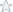Business Statistics with Computer Applications II MS 3043

The project requirements were as follows:

Project Requirements – Fall 2011 – Due: November 22, 2011
Business Statistics with Computer Applications II (MS 3043)
1. Go to this site: [link removed]
2. Determine which one of the three variables you want to be your
predictor variable, and which one you want to be your criterion variable.
3. Select 20 beers that you would like to include in your analysis—try not
to just pick the first 20 beers.
4. Conduct a Regression Analysis in Excel using only two variables (those
you selected in step 2).
5. Using your Excel output:
o If predicting calories, predict the calorie level for a beer with an
alcohol level of 4.95%, or 8.75 g of carbs.
o If predicting alcohol level, predict the alcohol level for a beer with
155 calories, or 9.25 g of carbs.
o If predicting carbs, predict the number of carbs for a beer with
5.15% alcohol, or 160 calories.
6. Show your work. Show the formula, and how you used the numbers
from Excel to make your prediction.
7. Include a printout of your Excel results. Highlight the slope and
intercept number you used for the calculation.
Requirements:
o Two-pages.
o Page 1: your calculations and predicted y value (step 3).
o Page 2: your Excel printout and the data list you used (step 4).
o 12 point font, double-spaced.
o Must be professional-looking (so, spell check and have a friend read it).

The first two sections of the answer concerning sample selection and Regression equation are reproduced below. You can purchase the full solutions to view them in their entirety.

Sample Selection

There were a total of 163 beers available for analysis, however, only a random sample of 20 will be used to develop the regression equation. This was done by assigning each beer a random number in EXCEL using the “Rand” function, and then picking the 20 beers with the smallest random numbers. This guaranteed that the selection of the beers was completely random and taken out of “human” hands

Regression Equation
Using the twenty selected beers a regression equation we developed using EXCEL predicting carbohydrates based on calories. The regression equation is:

CARBS =-1.6639+.0872*(Calories)

This model had an R-square of .706 indicating about 70% of the variability in carbohydrates are explained by the regression equation, and the calories of a beer have a significant positive association with carbs (p<.001).

Other Details about the Project/Assignment
Subjects: Statistics -> Regression Analysis
Topic: Regression Analysis in Excel using two variables
Level: College / University
Tags:

Business Statistics, Computer Applications, MS 3043, Regression Analysis, Excel, two variables

Price\$2.95

Math Genius
 Frederick Burke Member Since: March 2003 Customer Rating: 94.7% Projects Completed: 795 Total Earnings: *Private* +1 Ratings from clients: 115
Project Details
Subjects: Statistics -> Regression Analysis
Topic: Regression Analysis in Excel using two variables
Level: College / University
Tags:

Business Statistics, Computer Applications, MS 3043, Regression Analysis, Excel, two variables

Customer Reviews
Rated 2 Times
Rating( 4.5 / 5 Stars)

Reviews of the solutions left by other users
No customer has left a detailed review for these math solutions. Be the first one to purchase them and leave a review for others!

Not exactly what you are looking for?
We regularly update our math homework solutions library and are continually in the process of adding more samples and complete homework solution sets. If you do not find what you are looking for, just go ahead and place an order for a custom created homework solution. You can hire/pay a math genius to do your homework for you exactly to your specifications.